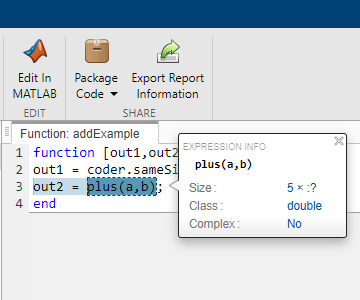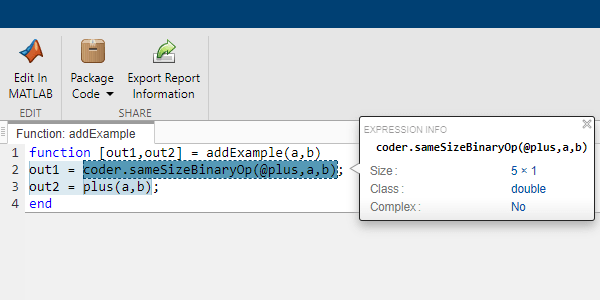# coder.sameSizeBinaryOp

Apply element-wise binary operations without implicit expansion

## Syntax

``result = coder.sameSizeBinaryOp(func_handle,u,v)``

## Description

example

````result = coder.sameSizeBinaryOp(func_handle,u,v)` performs an error check that the operands are of the same size and applies the binary operation or function specified by the function handle `func_handle` on the operands `u` and `v` without implicitly expanding them. The operands must be of the same size because this function does not allow scalar expansion.Use `coder.sameSizeBinaryOp` to disable implicit expansion for a specific binary operation or function. Disable implicit expansion to avoid automatic size change of output sizes, additional code generation, and performance variation. See Generate Code With Implicit Expansion Enabled, Optimize Implicit Expansion in Generated Code and Compatible Array Sizes for Basic Operations.To disable implicit expansion for all binary operations and functions within a specific function in the generated code, call `coder.noImplicitExpansionInFunction` within that function.```

## Examples

collapse all

Use `coder.sameSizeBinaryOp` to apply binary operations and functions where implicit expansion is not required.

Using `coder.sameSizeBinaryOp` ensures that any variable-size operands of compatible sizes are not expanded automatically. The generated code does not include additional code to enable the automatic expansion of the operands.

In this example, the `plus` function applies the operation with implicit expansion. The `coder.sameSizeBinaryOp` function applies the operation without implicit expansion.

`type addExample.m`
```function [out1,out2] = addExample(a,b) out1 = coder.sameSizeBinaryOp(@plus,a,b); out2 = plus(a,b); end ```

Define the input types.

```a_type = coder.typeof(1,[5 1]); b_type = coder.typeof(1,[5 inf]);```

Generate code for the function `addExample` by using this command.

`codegen addExample -args {a_type, b_type} -config:lib -report`
```Code generation successful: To view the report, open('codegen/lib/addExample/html/report.mldatx') ```

Compare Output Sizes

In the code generation report created in the previous step, place your cursor over the two operations.The size of the output from the `plus` operation is `5x:?` whereas the size of the output of `coder.sameSizeBinaryOp` is `5x1`.

The `plus` function implicitly expands its operands to the output size.

The `coder.sameSizeBinaryOp` function disables implicit expansion to prevent the automatic expansion of the operands.

Examine the Generated Code

`type codegen/lib/addExample/addExample.c`
```/* * File: addExample.c * * MATLAB Coder version : 5.4 * C/C++ source code generated on : 26-Feb-2022 10:44:03 */ /* Include Files */ #include "addExample.h" #include "addExample_emxutil.h" #include "addExample_types.h" /* Function Definitions */ /* * Arguments : const double a * const emxArray_real_T *b * double out1 * emxArray_real_T *out2 * Return Type : void */ void addExample(const double a, const emxArray_real_T *b, double out1, emxArray_real_T *out2) { const double *b_data; double *out2_data; int i; int i1; int loop_ub; b_data = b->data; for (i = 0; i < 5; i++) { out1[i] = a[i] + b_data[i]; } i = out2->size * out2->size; out2->size = 5; out2->size = b->size; emxEnsureCapacity_real_T(out2, i); out2_data = out2->data; loop_ub = b->size; for (i = 0; i < loop_ub; i++) { for (i1 = 0; i1 < 5; i1++) { int i2; i2 = i1 + 5 * i; out2_data[i2] = a[i1] + b_data[i2]; } } } /* * File trailer for addExample.c * * [EOF] */ ```

The code generated to calculate `out1` by using `coder.sameSizeBinaryOp` is seen in the first `for` loop in the function `addExample`. The code generated to calculate `out2` is seen below the first `for` loop. The generated code for `out1` is much smaller, as compared to the code generated to calculate `out2`.

The code generated for the `plus` function needs additional code to expand its operands.

This example shows how to generate code for binary operations and functions without implicitly expanding its operands.

The `coder.sameSizeBinaryOp` function applies the required operation and bypasses additional code generation and change in output size associated with implicit expansion.

This example highlights the difference between the code generated for `coder.sameSizeBinaryOp` and the code generated for the `minus` function.

Subtracting Two Operands With Implicit Expansion

For this code snippet, the generated code implicitly expands the output.

`type fooImpEx.m`
```function out = fooImpEx(a,b) out = b - a; end ```

Define the operand types.

`a = coder.typeof(1,[2 1])`
```a = coder.PrimitiveType 2×1 double Edit Type Object ```
`b = coder.typeof(1,[2 inf])`
```b = coder.PrimitiveType 2×:inf double Edit Type Object ```

Generate code for the function by running this command:

`codegen fooImpEx -config:lib -args {a,b}`
```Code generation successful. ```

The code generated for the function `fooImpEx` is shown here.

`type codegen/lib/fooImpEx/fooImpEx.c`
```/* * File: fooImpEx.c * * MATLAB Coder version : 5.4 * C/C++ source code generated on : 26-Feb-2022 10:47:07 */ /* Include Files */ #include "fooImpEx.h" #include "fooImpEx_emxutil.h" #include "fooImpEx_types.h" /* Function Definitions */ /* * Arguments : const double a * const emxArray_real_T *b * emxArray_real_T *out * Return Type : void */ void fooImpEx(const double a, const emxArray_real_T *b, emxArray_real_T *out) { const double *b_data; double *out_data; int i; int loop_ub; b_data = b->data; i = out->size * out->size; out->size = 2; out->size = b->size; emxEnsureCapacity_real_T(out, i); out_data = out->data; loop_ub = b->size; for (i = 0; i < loop_ub; i++) { int i1; out_data[2 * i] = b_data[2 * i] - a; i1 = 2 * i + 1; out_data[i1] = b_data[i1] - a; } } /* * File trailer for fooImpEx.c * * [EOF] */ ```

The generated code includes code to automatically expand the size of compatible operands.

Subtracting Two Same-Size Operands Without Implicit Expansion

This code snippet uses `coder.sameSizeBinaryOp` to apply the operation without using implicit expansion.

`type fooSameSize.m`
```function out = fooSameSize(a,b) out = coder.sameSizeBinaryOp(@minus,b,a); end ```

Generate code for the function by running this command:

`codegen fooSameSize -config:lib -args {a,b}`
```Code generation successful. ```

The code generated for the function `fooImpEx` is shown here.

`type codegen/lib/fooSameSize/fooSameSize.c`
```/* * File: fooSameSize.c * * MATLAB Coder version : 5.4 * C/C++ source code generated on : 26-Feb-2022 10:47:11 */ /* Include Files */ #include "fooSameSize.h" #include "fooSameSize_types.h" /* Function Definitions */ /* * Arguments : const double a * const emxArray_real_T *b * double out * Return Type : void */ void fooSameSize(const double a, const emxArray_real_T *b, double out) { const double *b_data; b_data = b->data; out = b_data - a; out = b_data - a; } /* * File trailer for fooSameSize.c * * [EOF] */ ```

In this case, the variable `out` is fixed-size and the code generated for the operation applied by the `coder.sameSizeBinaryOp` function does not expand the operands. The generated function `fooSameSize` does not contain additional loops to increase the size of the operands.

## Input Arguments

collapse all

Binary function to apply, specified as a function handle, `func_handle` must be a binary (two-input) element-wise function of the form `C = func_handle(u,v)` that accepts arrays `u` and `v` with the same-size. Apply these binary functions without implicit expansion by using `coder.sameSizeBinaryOp`:

FunctionSymbolDescription
`plus``+`Plus
`minus``-`Minus
`times``.*`Array multiply
`rdivide``./`Right array divide
`ldivide``.\`Left array divide
`power``.^`Array power
`eq``==`Equal
`ne``~=`Not equal
`gt``>`Greater than
`ge``>=`Greater than equal to
`lt``<`Less than
`le``<=`Less than equal to
`and``&`Element-wise logical AND
`or``|`Element-wise logical OR
`xor`N/ALogical exclusive OR
`bitand`N/ABit-wise AND
`bitor`N/ABit-wise OR
`bitxor`N/ABit-wise XOR
`max`N/ABinary maximum
`min`N/ABinary minimum
`mod`N/AModulus after division
`rem`N/ARemainder after division
`atan2`N/AFour-quadrant inverse tangent; result in radians
`atan2d`N/AFour-quadrant inverse tangent; result in degrees
`hypot`N/ASquare root of sum of squares

Example: ```result = coder.sameSizeBinaryOp(@plus, u, v);```

Data Types: `function_handle`

Input array, specified as scalar, vector, matrix, or multidimensional array. Inputs `u` and `v` must be of the same sizes.

Data Types: `single` | `double` | `int8` | `int16` | `int32` | `int64` | `uint8` | `uint16` | `uint32` | `uint64` | `logical` | `char` | `string` | `struct` | `table` | `cell` | `function_handle` | `categorical` | `datetime` | `duration` | `calendarDuration` | `fi`
Complex Number Support: Yes

Input array, specified as scalar, vector, matrix, or multidimensional array. Inputs `u` and `v` must be of the same sizes.

Data Types: `single` | `double` | `int8` | `int16` | `int32` | `int64` | `uint8` | `uint16` | `uint32` | `uint64` | `logical` | `char` | `string` | `struct` | `table` | `cell` | `function_handle` | `categorical` | `datetime` | `duration` | `calendarDuration` | `fi`
Complex Number Support: Yes

## Version History

Introduced in R2021b